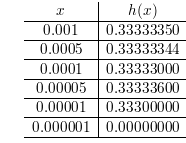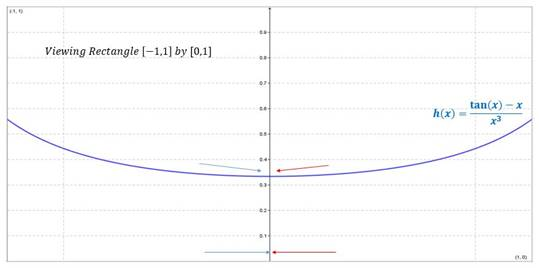# To evaluate the given function for given values.### Single Variable Calculus: Concepts...

4th Edition
James Stewart
Publisher: Cengage Learning
ISBN: 9781337687805### Single Variable Calculus: Concepts...

4th Edition
James Stewart
Publisher: Cengage Learning
ISBN: 9781337687805

#### Solutions

Chapter 2.2, Problem 30E

a.

To determine

## To evaluate the given function for given values.

Expert Solution

h(1)=0.557408h(0.5)=0.37042h(0.1)=0.334672h(0.05)=0.333667h(0.01)=0.333347h(0.05)=0.333337

### Explanation of Solution

Given:

The given function is h(x)=tanxxx3

Calculation:

Substituting the following values in the given function as follows-

h(x)=tanxxx3h(1)=0.557408h(0.5)=0.37042h(0.1)=0.334672h(0.05)=0.333667h(0.01)=0.333347h(0.05)=0.333337

b.

To determine

Expert Solution

13

### Explanation of Solution

Given:

The given function is limx0tanxxx3

Calculation:

limx0tanxxx3limx0tanxxx3=0.33333=13

c.

To determine

Expert Solution

No.

### Explanation of Solution

Given:

The given function is limx0tanxxx3

Calculation:

The following is the table of all smaller values-The guess in part (b) does not seem to be correct.

d.

To determine

Expert Solution

### Explanation of Solution

Given:

The given function is limx0tanxxx3

Calculation:

The following are the graphs-In part (C), it was observed that limx0tanxxx3=0

From the above graphs the limit does not approach to a specific value, hence the limit does not exist.

### Have a homework question?

Subscribe to bartleby learn! Ask subject matter experts 30 homework questions each month. Plus, you’ll have access to millions of step-by-step textbook answers!